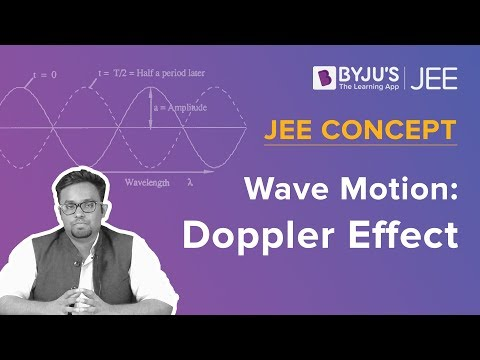# CBSE Class 12 Physics Notes Chapter 10 Wave Optics

## What is the Huygens Principle?

According to this principle, every point in the wavefront is a potential source of secondary waves and eventually, adds up to give us the wavefront. When the light’s speed does not depend on the direction, then the secondary waves are spherical in nature.

Related Topics:

What is Optics

Important Questions on Wave Optics

Revision Notes for Waves Optics

NCERT Exemplars for Wave Optics

NCERT Solutions for Wave Optics

### Principle of Superposition

This principle is applicable whenever there are multiple sources of light illuminating a particular point. There lies an interference term to the sum of the individual intensities whenever we consider the intensity of light due to its point sources. But this source works when they have the same frequency and have stability in the phase difference.

### Plane Polarised light

The light which gets emitted from a polaroid is called polarised light which means, it only transmits one component of light the one which is parallel in nature. Two maxima and minima can be seen when there a second polaroid having an axis turn of

$$\begin{array}{l}2\pi\end{array}$$
is used to view the light. Polarised light can also be seen when reflected at the Brewster angle and by scattering through
$$\begin{array}{l}\pi/2\end{array}$$
in the atmosphere of the earth.

### Properties of waves

Waves when released from a point source, scatters in all directions, but the light is seen to be travelling in narrow rays. All the aspects and behaviour of light has been explained in the experiments of Fresnel, Young, and Huygens.

According to Young’s experiment, the key feature about the wave is the interference of amplitude occurring due to various sources and can be constructive and destructive in nature.

The limit of the ability of a microscope and telescopes of differentiating small objects is defined by the wavelength of the light. The limits of ray optics are defined by diffraction.

The polarisation phenomena are specific to light waves or transverse waves but interference and diffraction also occur for longitudinal waves such as the sound waves in air.

#### To Know About Doppler Effect, Watch The Below Video:To know more about wave optics and related topics, keep visiting BYJU’S.

### Important Questions

1. The wavelength of monochromatic light is 600 nm and is being incident from air to the water surface. Find out the frequency, wavelength, and speed of (a) refracted (b) reflected light? Refractive index of water is assumed to 1.33
2. The slit and the screen are positioned as 0.30mm and 2 m apart. The distance between the fourth bright fringe and the central fringe is 2 cm. Find out the light’s wavelength?
3. The angular width of a fringe is 0.5° on a screen which is positioned 2 m away in a double-slit experiment. The wavelength of the used light 500 nm. Find out the angular width of the fringe when the entire setup is under water. Assume refractive index to be 5/4?

## Frequently asked Questions on CBSE Class 12 Physics Notes Chapter 10: Wave Optics

### What is ‘Superposition’?

The superposition principle states that when two or more waves overlap in space, the resultant disturbance is equal to the algebraic sum of the individual disturbances.

### What is the ‘Huygen’s Principle’?

Huygens principle states that every point on a wavefront is in itself the source of spherical wavelets. The sum of these spherical wavelets forms the wavefront.

### What are the properties of waves?

There are many properties that scientists use to describe waves. They include amplitude, frequency, period, wavelength, speed, and phase.